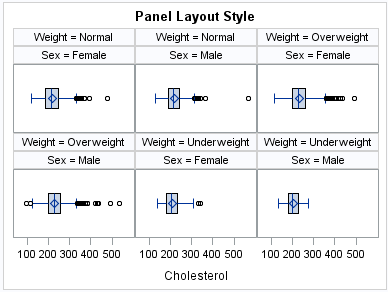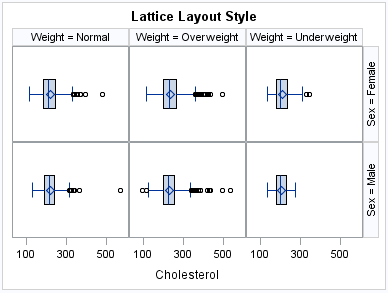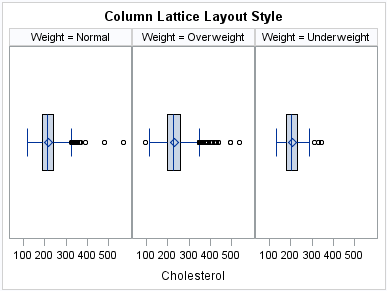# Concepts: SGPANEL Procedure

## Panel Creation

The SGPANEL procedure has a required PANELBY statement that is used to define the classifier variables for the panel. This statement must be specified before any plot, axis, or legend statement or else an error occurs. You can use options in the PANELBY statement to control the attributes of the panel. For example, you can use the COLUMNS= option to specify the number of columns in the panel.
SGPANEL can use four different layouts, which are specified by the LAYOUT= option in the PANELBY statement. The layout determines how your classifier variables are used to create the panel, and also affects the number of classifier variables that you can specify.
The default layout is PANEL. With this layout, you can specify any number of classifier variables. The graph cells in the panel are arranged automatically, and the classifier values are displayed above each graph cell in the panel. When you specify multiple classifier variables, the order of the classifier variables determines how the graph cells are sorted.
Example of the PANEL LayoutAnother layout is called LATTICE. This layout requires exactly two classifier variables. The values of the first variable are assigned as columns, and the values of the second variable are assigned as rows. The classifier values are displayed above the columns and to the right side of the rows.
Example of the LATTICE LayoutTwo additional layouts are available, which are called COLUMNLATTICE and ROWLATTICE. These layouts require exactly one classifier variable. The values of the classifier variable are assigned as cells in a single row or column.
Example of the COLUMNLATTICE LayoutIf you have a large number of classifier variables, then the best method for creating a panel is to choose one or two classifiers for the PANELBY statement. Then specify the remaining variables in a BY statement. This method maximizes the space for the plots and generates results that are easier to interpret.

## Plot Type Compatibility

Each graph cell in your panel contains one or more plots, and there are four basic types of plots that you can create with the SGPANEL procedure.
Basic plots
scatter, series, step, band, bubble, high-low, needle plots, and vector plots
Fit and confidence plots
loess, regression, and penalized B-spline curves
Distribution plots
box plots, histograms, normal density curves, and kernel density estimates
Categorization plots
dot plots, bar charts, and line plots
Not all of the plot types can be used together in the same PROC SGPANEL step. The following table shows which of the plot types can be used together:
Plot Type Compatibility
Basic
Fit and Confidence
Distribution
Categorization
Basic
x
x
Fit and Confidence
x
x
Distribution
x
Categorization
x
Note: Box plots cannot be combined with any other plot types. Box plots can be overlaid with other box plots. However, overlaid box plots must have the same category variables.
If you submit a PROC SGPANEL step that combines two incompatible plot statements, then an error appears in the log.
The SGPANEL procedure draws the plots in your graph in the same order that you specify the plot statements. Because of this, it is important to consider the order of your plot statements so that your plots do not obscure one another. For example, if you specify a BAND statement after a SCATTER statement, then the band plot might obscure the markers in your scatter plot. You can also avoid obscuring your data by using the TRANSPARENCY= option to make your plots partially transparent.

## Plot Axes

The SGPANEL procedure contains two statements that enable you to change the type and appearance for the axes of the graph cells in your panel: COLAXIS and ROWAXIS.
By default, the type of each axis is determined by the types of plots that use the axis and the data that is applied to the axis.
The SGPANEL procedure supports the following axis types:
Discrete
The axis contains independent data values rather than a range of numeric values. Each distinct value is represented by a tick mark. Discrete is the default axis type for character data.
Linear
The axis contains a linear range of numeric values. Linear is the default axis type for numeric data.
Logarithmic
The axis contains a logarithmic range of values. The logarithmic axis type is not used as a default.
Time
The axis contains a range of time values. Time is the default axis type for data that uses a SAS time, date, or datetime format.
Some types of plot do not support all of the axis types. For example, needle plots cannot use a discrete vertical axis. See the documentation for each plot statement to determine whether any axis type restrictions apply.

## Panel Legends

The SGPANEL procedure creates a legend automatically based on the plot statements and options that you specify. The automatic legend functionality determines which information is likely to be useful in the legend. You can override this behavior by defining your own legend with the KEYLEGEND statement or by specifying the NOAUTOLEGEND option in the PROC SGPANEL statement.
You can specify the labels that represent your plots in the legend by using the LEGENDLABEL= option in the corresponding plot statements.
You can create customized legends by using one or more KEYLEGEND statements. You can specify which plot statements are assigned to the legend, and use options to control the title, location, and border of the legend. For more information, see .

## Automatic Differentiation of Visual Attributes

Depending on the plots and options that you specify, the SGPANEL procedure can automatically assign different style attributes to the plots in your graph. For example, if you specify two series plots, then each series plot automatically uses a different line pattern and line color by default. If different attributes are not assigned by default, you can force the procedure to assign different style attributes by using the CYCLEATTRS option in the PROC SGPANEL statement. You can also disable automatic attribute differentiation by using the NOCYCLEATTRS option in the PROC SGPANEL statement.